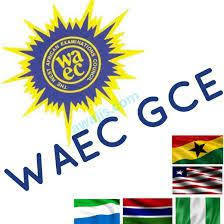# 2018 Waec Gce Practical Physics Answer / Expo / RunzWAEC GCE Physics Specimen 2018

QUESTION 1
Uniform metre rule;
Knife edge; 100g mass
labelled N; 50g mass

QUESTION 2
Beaker (0-500cm3);
Thermometer (0-110
degree C);
Measuring cylinder
(0-500cm3);
50g mass;

A pair of tongs; Bunsen burner (source of heat);
Tripod stand; Water, wire gauze, stirrer; Stop clock/watch; Flat plank (10cm x 15cm);

QUESTION 3
Variable d.c power supply;
(Battery rack capable of containing five 1.5V dry cells in series);
2 ohms standard resistor; Key;
Ammeter (0-3A);
Voltmeter (0-5V);
Six connecting wires.

Conservation of energy
The law of conservation of energy stated that energy cannot be created or destroy but it can be transformed from one form to another. This implies that when energy is converted from one form to another, no energy is lost or created during the conversion process.

Dalton's law of partial pressures
Dalton's law (also called Dalton's law of partial pressures) states that the total pressure exerted by the mixture of non-reactive gases is equal to the sum of the partial pressures of individual gases.

Ohm's law
Ohm's law emphasis the principle that the electric current passing through a conductor is directly proportional to the potential difference across it, provided that the temperature remains constant. The constant of proportionality is the resistance of the conductor

Hooke's law
Hooke's law is a principle of physics that states that the force F needed to extend or compress a spring by some distance X is proportional to that distance.

Ideal Gas Law
An ideal gas is defined as one in which all collisions between atoms or molecules are perfectly eleastic and in which there are no intermolecular attractive forces.

Snell's Law
Snell's Law relates the indices of refraction n of the two media to the directions of propagation in terms of the angles to the normal.

Law of Refraction
Law of Refraction stated that When light travels from one medium to another, it generally bends.

Tabulate
s/n 1,2,3,4,5
D(cm) 25,35,50,65,80
I(A) 1.99,1.75,1.55,1.44,1.36
I^(A^-1) 0.530,0.571,0.645,0.694,0.735
Lo(j^) -0.2984,-0.2434,-0.1904,-0.1586,-0.1337

3iii)

3viiii)
slope Dvertical/Dhorizontal
=1.41-1.00/80-25
=0.4/55
S=0.00727

3x)
from the graph C=32cm

3xi)
K=c/2=33/2=16cm

3xii)
-precautions-
take any two
i)I removed key when reading is not taking
ii)I ensured tight connections at the terminals
iii)I avoided parallax error when taking reading from the ammeter.

3bii)
i)length – longer wires have greater resistance.
ii)thickness – smaller diameter wires have greater resistance.

2ai)
fo=15cm

2av)
a=60.00cm
b=20.00cm
Hence L=a/b=60.00/20.00
L=3

2avi)
TABULATE
S/N:1,2,3,4,5
b(cm):20.00,25.00,35.00,40.00
a(cm):60.00,37.50,30.00,26.25,24.00
L=a/b:3.00,1.50,1.00,0.75,0.60

2avii)
Slope=Change in L/Change in a
=(3-0.25)/(60-18.6)
=2.75/41.4
=0.006642
2aviii)
S^-1=1/S
=(1/0.0066425cm)
S=15.05
S=15cm

2aix)
PRECAUTIONS
-I ensured that all apparatus are in straight line
-I avoided error due to parallax when reading the metre rule
-I avoided zero error on the metre rule

2bi)
u=10cm
f=15cm
Using 1/v+1/u=1/f
1/f-1/u=1/v
1/v=1/15-1/10
1/v=(2-3)/30
1/v=-1/30
v=-30cm
The characteristics of image formed are:
-It is virtual
-It is enlarged and magnified ie twice or two times as big as the object m=2

2bii)
The concave mirror mounted in its holder is moved to and fro in front of the screen until a sharp image of the cross wire of the ray box is formed on the screen adjacent of the object.The distance between the mirror and the screen was measured as 30cm since the radius of curvature r=2fo then half is distance

2bi)
The characteristics of imaged formed are :
i)It is virtual
ii) It is enlarged or magnified i.e. twice or two times bigger as the object(m=2)

1)
Tabulate
S/n 1,2,3,4,5
L(cm) 90.00,80.00,70.00,60.00,50.00
T(s) 38.30,35.40,33.40,29.60,27.50
T=t/20(s) 1.92,1.77,1.67,1.48,1.38
sqrL(cm^1/2) 9.49,8.94,8.37,7.75,7.07

1vii)

1ix)
slope (s) = D vertical/D horizontal
= 1.90-155/9.49-775
=0.35/1.75
s=0.201

1x)
g=4^2/s^2
=4*(3.142)^2/(0.201)^2
=977.4cm1s^2

1xii)
-precautions-
Take Any Two
i)I avoided conical oscillation
ii)I avoided drought
iii)I avoided parallax error in taking reading from the metre rule
iv)I avoided zero error in taking reading from the stop watch

1aix )
i ) I avoided parallax error in reading clock / weight balance
ii) I ensue repeated readings are taken to avoid random error
========================================

3x)
i ) I would ensure that the terminal are well tightened to avoid partial contact

ii) I would obey the connection of positive to positive to negative when connecting to circuit

3a(vi)
¶ Ensure tight connection s.
¶ Avoid error due to parallax when reading the voltmeter

3b
(i) ¶ Length of wire
¶ nature of wire

(ii) Total resistance,
R = 3 + (3×3)/(3+3)
R = 4.5 ohms

Current, I = V/R
I = 6/4.5
I = 1.33 A

Atlas team
[9/5, 10:07 AM] ‪+234 808 669 7392‬: RC ®)

= 1.gov, N, 2-15V
V=2.3V V4 2.40V
V5 = 2.5V

11.00 1.90 1-on 6.5263 1.900
2.00 2.15 0.500 0.468 1.07
3-00 2:30 0.333 0.43470-760
4.000 2.40 0.250 0.41670-5999
5 15.00 2.50 0.20 0.400 0.500

I would ensure tight connection during the experiment.
I will ensure the key removed when the court is not used

NB:
Measurements on *Questions 1 and 2* cannot be taken accurately through electronic copy of the question paper.

Only question 3 can be done as it doesn't involve measurement

(3a)
R ohms
R1 = 1ohms R3 = 3ohms
R2 = 2ohms R4 = 4ohms
R5 = 5ohms
V1 = 1.90v
V2 = 2.15v
V3 = 2.3v
V4 = 2.40v
V5 = 2.5v

In a tabular form
Under S/N
1, 2, 3, 4, 5

Under R(ohms )
1.00, 2.00, 3.00, 4.00, 5.00

Under V(v)
1.90, 2.15, 2.30, 2.40, 2.50

Under R^-1(ohms^-1)
1.000, 5.000, 0.333, 0.250, 0.200

Under V^-1(V^-1)
0.5263, 0.465, 0.4347, 0.4167, 0.400

Under R^-1/V^-1
1.900, 1.07, 0.768, 0.5999, 0.500

(3avi)
(i) Ensure tight connections.
(ii) Avoid error due to parallax when reading the voltmeter

(3b)
(i) Length of wire
(ii) Nature of wire

(3bii)
Total resistance,
R = 3 + (3×3)/(3+3)
R = 4.5 ohms
Current, I = V/R
I = 6/4.5
I = 1.33 A